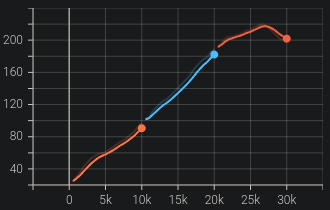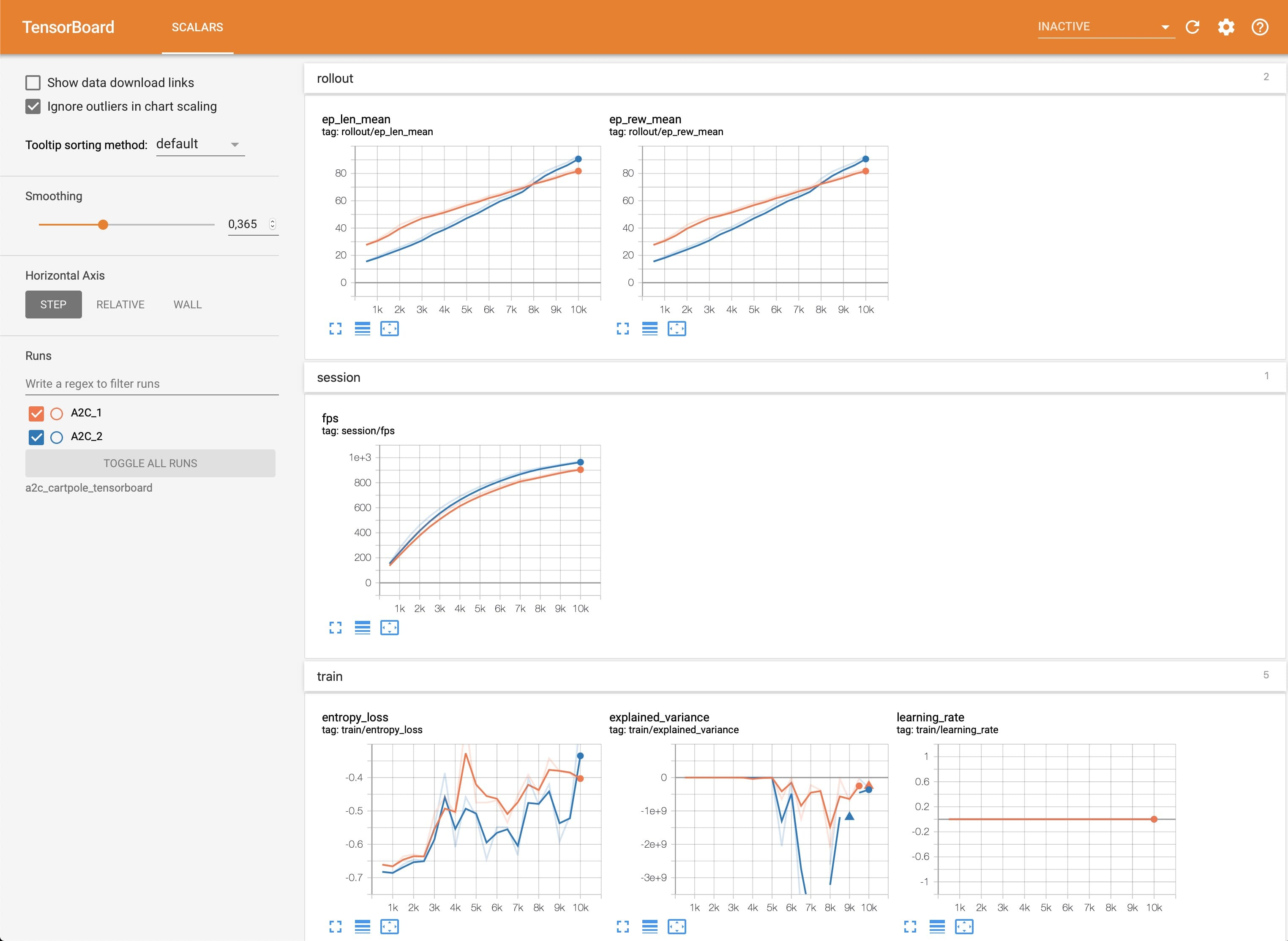# Tensorboard Integration

## Basic Usage

To use Tensorboard with stable baselines3, you simply need to pass the location of the log folder to the RL agent:

```from stable_baselines3 import A2C

model = A2C("MlpPolicy", "CartPole-v1", verbose=1, tensorboard_log="./a2c_cartpole_tensorboard/")
model.learn(total_timesteps=10_000)
```

You can also define custom logging name when training (by default it is the algorithm name)

```from stable_baselines3 import A2C

model = A2C("MlpPolicy", "CartPole-v1", verbose=1, tensorboard_log="./a2c_cartpole_tensorboard/")
model.learn(total_timesteps=10_000, tb_log_name="first_run")
# Pass reset_num_timesteps=False to continue the training curve in tensorboard
# By default, it will create a new curve
# Keep tb_log_name constant to have continuous curve (see note below)
model.learn(total_timesteps=10_000, tb_log_name="second_run", reset_num_timesteps=False)
model.learn(total_timesteps=10_000, tb_log_name="third_run", reset_num_timesteps=False)
```

Note

If you specify different `tb_log_name` in subsequent runs, you will have split graphs, like in the figure below. If you want them to be continuous, you must keep the same `tb_log_name` (see issue #975). And, if you still managed to get your graphs split by other means, just put tensorboard log files into the same folder.Once the learn function is called, you can monitor the RL agent during or after the training, with the following bash command:

```tensorboard --logdir ./a2c_cartpole_tensorboard/
```

Note

You can find explanations about the logger output and names in the Logger section.

you can also add past logging folders:

```tensorboard --logdir ./a2c_cartpole_tensorboard/;./ppo2_cartpole_tensorboard/
```

It will display information such as the episode reward (when using a `Monitor` wrapper), the model losses and other parameter unique to some models.## Logging More Values

Using a callback, you can easily log more values with TensorBoard. Here is a simple example on how to log both additional tensor or arbitrary scalar value:

```import numpy as np

from stable_baselines3 import SAC
from stable_baselines3.common.callbacks import BaseCallback

model = SAC("MlpPolicy", "Pendulum-v1", tensorboard_log="/tmp/sac/", verbose=1)

class TensorboardCallback(BaseCallback):
"""
Custom callback for plotting additional values in tensorboard.
"""

def __init__(self, verbose=0):
super().__init__(verbose)

def _on_step(self) -> bool:
# Log scalar value (here a random variable)
value = np.random.random()
self.logger.record("random_value", value)
return True

model.learn(50000, callback=TensorboardCallback())
```

Note

If you want to log values more often than the default to tensorboard, you manually call `self.logger.dump(self.num_timesteps)` in a callback (see issue #506).

## Logging Images

TensorBoard supports periodic logging of image data, which helps evaluating agents at various stages during training.

Warning

To support image logging pillow must be installed otherwise, TensorBoard ignores the image and logs a warning.

Here is an example of how to render an image to TensorBoard at regular intervals:

```from stable_baselines3 import SAC
from stable_baselines3.common.callbacks import BaseCallback
from stable_baselines3.common.logger import Image

model = SAC("MlpPolicy", "Pendulum-v1", tensorboard_log="/tmp/sac/", verbose=1)

class ImageRecorderCallback(BaseCallback):
def __init__(self, verbose=0):
super().__init__(verbose)

def _on_step(self):
image = self.training_env.render(mode="rgb_array")
# "HWC" specify the dataformat of the image, here channel last
# (H for height, W for width, C for channel)
# See https://pytorch.org/docs/stable/tensorboard.html
# for supported formats
self.logger.record("trajectory/image", Image(image, "HWC"), exclude=("stdout", "log", "json", "csv"))
return True

model.learn(50000, callback=ImageRecorderCallback())
```

## Logging Figures/Plots

TensorBoard supports periodic logging of figures/plots created with matplotlib, which helps evaluating agents at various stages during training.

Warning

To support figure logging matplotlib must be installed otherwise, TensorBoard ignores the figure and logs a warning.

Here is an example of how to store a plot in TensorBoard at regular intervals:

```import numpy as np
import matplotlib.pyplot as plt

from stable_baselines3 import SAC
from stable_baselines3.common.callbacks import BaseCallback
from stable_baselines3.common.logger import Figure

model = SAC("MlpPolicy", "Pendulum-v1", tensorboard_log="/tmp/sac/", verbose=1)

class FigureRecorderCallback(BaseCallback):
def __init__(self, verbose=0):
super().__init__(verbose)

def _on_step(self):
# Plot values (here a random variable)
figure = plt.figure()
# Close the figure after logging it
self.logger.record("trajectory/figure", Figure(figure, close=True), exclude=("stdout", "log", "json", "csv"))
plt.close()
return True

model.learn(50000, callback=FigureRecorderCallback())
```

## Logging Videos

TensorBoard supports periodic logging of video data, which helps evaluating agents at various stages during training.

Warning

To support video logging moviepy must be installed otherwise, TensorBoard ignores the video and logs a warning.

Here is an example of how to render an episode and log the resulting video to TensorBoard at regular intervals:

```from typing import Any, Dict

import gymnasium as gym
import torch as th

from stable_baselines3 import A2C
from stable_baselines3.common.callbacks import BaseCallback
from stable_baselines3.common.evaluation import evaluate_policy
from stable_baselines3.common.logger import Video

class VideoRecorderCallback(BaseCallback):
def __init__(self, eval_env: gym.Env, render_freq: int, n_eval_episodes: int = 1, deterministic: bool = True):
"""
Records a video of an agent's trajectory traversing ``eval_env`` and logs it to TensorBoard

:param eval_env: A gym environment from which the trajectory is recorded
:param render_freq: Render the agent's trajectory every eval_freq call of the callback.
:param n_eval_episodes: Number of episodes to render
:param deterministic: Whether to use deterministic or stochastic policy
"""
super().__init__()
self._eval_env = eval_env
self._render_freq = render_freq
self._n_eval_episodes = n_eval_episodes
self._deterministic = deterministic

def _on_step(self) -> bool:
if self.n_calls % self._render_freq == 0:
screens = []

def grab_screens(_locals: Dict[str, Any], _globals: Dict[str, Any]) -> None:
"""
Renders the environment in its current state, recording the screen in the captured `screens` list

:param _locals: A dictionary containing all local variables of the callback's scope
:param _globals: A dictionary containing all global variables of the callback's scope
"""
screen = self._eval_env.render(mode="rgb_array")
# PyTorch uses CxHxW vs HxWxC gym (and tensorflow) image convention
screens.append(screen.transpose(2, 0, 1))

evaluate_policy(
self.model,
self._eval_env,
callback=grab_screens,
n_eval_episodes=self._n_eval_episodes,
deterministic=self._deterministic,
)
self.logger.record(
"trajectory/video",
Video(th.ByteTensor([screens]), fps=40),
exclude=("stdout", "log", "json", "csv"),
)
return True

model = A2C("MlpPolicy", "CartPole-v1", tensorboard_log="runs/", verbose=1)
video_recorder = VideoRecorderCallback(gym.make("CartPole-v1"), render_freq=5000)
model.learn(total_timesteps=int(5e4), callback=video_recorder)
```

## Logging Hyperparameters

TensorBoard supports logging of hyperparameters in its HPARAMS tab, which helps comparing agents trainings.

Warning

To display hyperparameters in the HPARAMS section, a `metric_dict` must be given (as well as a `hparam_dict`).

Here is an example of how to save hyperparameters in TensorBoard:

```from stable_baselines3 import A2C
from stable_baselines3.common.callbacks import BaseCallback
from stable_baselines3.common.logger import HParam

class HParamCallback(BaseCallback):
"""
Saves the hyperparameters and metrics at the start of the training, and logs them to TensorBoard.
"""

def _on_training_start(self) -> None:
hparam_dict = {
"algorithm": self.model.__class__.__name__,
"learning rate": self.model.learning_rate,
"gamma": self.model.gamma,
}
# define the metrics that will appear in the `HPARAMS` Tensorboard tab by referencing their tag
# Tensorbaord will find & display metrics from the `SCALARS` tab
metric_dict = {
"rollout/ep_len_mean": 0,
"train/value_loss": 0.0,
}
self.logger.record(
"hparams",
HParam(hparam_dict, metric_dict),
exclude=("stdout", "log", "json", "csv"),
)

def _on_step(self) -> bool:
return True

model = A2C("MlpPolicy", "CartPole-v1", tensorboard_log="runs/", verbose=1)
model.learn(total_timesteps=int(5e4), callback=HParamCallback())
```

## Directly Accessing The Summary Writer

If you would like to log arbitrary data (in one of the formats supported by pytorch), you can get direct access to the underlying SummaryWriter in a callback:

Warning

This is method is not recommended and should only be used by advanced users.

Note

If you want a concrete example, you can watch how to log lap time with donkeycar env, or read the code in the RL Zoo. You might also want to take a look at issue #1160 and issue #1219.

```from stable_baselines3 import SAC
from stable_baselines3.common.callbacks import BaseCallback
from stable_baselines3.common.logger import TensorBoardOutputFormat

model = SAC("MlpPolicy", "Pendulum-v1", tensorboard_log="/tmp/sac/", verbose=1)

class SummaryWriterCallback(BaseCallback):

def _on_training_start(self):
self._log_freq = 1000  # log every 1000 calls

output_formats = self.logger.output_formats
# Save reference to tensorboard formatter object
# note: the failure case (not formatter found) is not handled here, should be done with try/except.
self.tb_formatter = next(formatter for formatter in output_formats if isinstance(formatter, TensorBoardOutputFormat))

def _on_step(self) -> bool:
if self.n_calls % self._log_freq == 0:
# You can have access to info from the env using self.locals.
# for instance, when using one env (index 0 of locals["infos"]):
# lap_count = self.locals["infos"]["lap_count"]